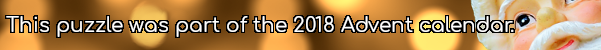mscroggs.co.uk
mscroggs.co.uksubscribe

# Advent calendar 2018

## 21 DecemberPut the digits 1 to 9 (using each digit exactly once) in the boxes so that the sums are correct. The sums should be read left to right and top to bottom ignoring the usual order of operations. For example, 4+3×2 is 14, not 10. Today's number is the smallest number you can make using the digits in the red boxes.
 + ÷ = 2 × + - × - = 31 + + - - × = 42 =37 =13 =-2
Tags: numbers, grids

## Archive

Show me a random puzzle
▼ show ▼
© Matthew Scroggs 2019